• 用C语言来模拟操作系统存储管理，主要包括：分页存储管理，分段存储管理，段页式存储管理.
• 操作系统 存储管理实验报告 有实验报告中要有的全部资料的 ~~~
• 课程名称 计算机操作系统 实验名称 存储管理实验 实验类型 验证 设计 综合 创新 【实验目的】 实验目的： 通过模拟实现请求页式存储管理的几种基本页面置换算法，了解虚拟存储技术的特点，掌握虚 拟存储请求页式...
华中农业大学  学生实验报告
课程名称	计算机操作系统	实验名称	存储管理实验	 实验类型	验证  设计
综合  创新
【实验目的】
实验目的：

通过模拟实现请求页式存储管理的几种基本页面置换算法，了解虚拟存储技术的特点，掌握虚 拟存储请求页式存储管理中几种基本页面置换算法的基本思想和实现过程，并比较它们的效率。
实验要求：掌握五种存储管理算法
1）最佳淘汰算法（OPT）
2）先进先出的算法（FIFO）
3）最近最久未使用算法（LRU）
4）最不经常使用算法（LFU）
5）最近未使用算法（NUR）
熟悉内存自由空闲队列的分配策略及熟悉内存分区的回收原则及实现过程
【实验内容】
设计一个虚拟存储区和内存工作区，并使用下述算法计算访问命中率。
1）最佳淘汰算法（OPT）
2）先进先出的算法（FIFO）
3）最近最久未使用算法（LRU）
4）最不经常使用算法（LFU）
5）最近未使用算法（NUR）
命中率＝１－页面失效次数／页地址流长度
模拟内存的动态分配和回收，并编程实现。
【实验环境】（含主要设计设备、器材、软件等）
Pc电脑一台

【实验步骤、过程】（含原理图、流程图、关键代码，或实验过程中的记录、数据等）
实验步骤：
程序说明及实现:
1.
先进先出算法：原理是优先淘汰最早进入内存的页面，FIFO 算法是最简单的页面置换算法。FIFO 页面置换算法为每个页面记录了调到内存的时间，即必须置换页面时会选择最旧的页面。“FIFO 算法当进程分配到的页面数增加时，缺页中断的次数可能增加也可能减少”。 FIFO 算法基于队列实现，不是堆栈类算法。注意，并不需要记录调入页面的确切时间，可以创建一个 FIFO 队列，来管理所有的内存页面。置换的是队列的首个页面。当需要调入页面到内存时，就将它加到队列的尾部。FIFO 页面置换算法易于理解和编程。然而，它的性能并不总是十分理想：其一，所置换的页面可以是很久以前使用过但现已不再使用的初始化模块。其二，所置换的页面可以包含一个被大量使用的变量，它早就初始化了，但仍在不断使用。
2.
最近最久未被使用算法：即淘汰最近没有使用的页面，选择最近最长时间未访问过的页面予以淘汰，它认为过去一段时间内未访问过的页面，在最近的将来可能也不会被访问。该算法为每个页面设置一个访问字段，来记录页面自上次被访问以来所经历的时间，淘汰页面时选择现有页面中值最大的予以淘汰。
3.
最近未使用算法：当某一页首次装入主存时，该帧的使用位设置为1;当该页随后再被访问到时，它的使用位也被置为1。对于页替换算法，用于替换的候选帧集合看做一个循环缓冲区，并且有一个指针与之相关联。当某一页被替换时，该指针被设置成指向缓冲区中的下一帧。当需要替换一页时，操作系统扫描缓冲区，以查找使用位被置为0的一帧。每当遇到一个使用位为1的帧时，操作系统就将该位重新置为0；如果在这个过程开始时，缓冲区中所有帧的使用位均为0，则选择遇到的第一个帧替换；如果所有帧的使用位均为1,则指针在缓冲区中完整地循环一周，把所有使用位都置为0，并且停留在最初的位置上，替换该帧中的页。由于该算法循环地检查各页面的情况，故称为 CLOCK 算法，又称为最近未用( Not Recently Used, NRU )算法。
4.
最佳置换算法：当要调入一页而必须淘汰旧页时，应该淘汰以后不再访问的页，或距最长时间后要访问的页面。它所产生的缺页数最少，然而，却需要预测程序的页面引用串，这是无法预知的，不可能对程序的运行过程做出精确的断言，不过此理论算法可用作衡量各种具体算法的标准。
5.最不经常使用置换算法：最不经常使用（LFU）页面置换算法要求置换具有最小计数的页面。这种选择的原因是，积极使用的页面应当具有大的引用计数。然而，当一个页面在进程的初始阶段大量使用但是随后不再使用时，会出现问题。由于被大量使用，它有一个大的计数，即使不再需要却仍保留在内存中。一种解决方案是，定期地将计数右移 1 位，以形成指数衰减的平均使用计数。
FF首次适应算法（First Fit）：该算法从空闲分区链首开始查找，直至找到一个能满足其大小要求的空闲分区为止。然后再按照作业的大小，从该分区中划出一块内存分配给请求者，余下的空闲分区仍留在空闲分区链中。特点： 该算法倾向于使用内存中低地址部分的空闲区，在高地址部分的空闲区很少被利用，从而保留了高地址部分的大空闲区。显然为以后到达的大作业分配大的内存空间创造了条件。
最佳适应算法（Best Fit）：该算法总是把既能满足要求，又是最小的空闲分区分配给作业。为了加速查找，该算法要求将所有的空闲区按其大小排序后，以递增顺序形成一个空白链。这样每次找到的第一个满足要求的空闲区，必然是最优的。
最坏适应算法（Worst Fit）:最坏适应算法是将输入的作业放置到主存中与它所需大小差距最大的空闲区中。空闲区大小由大到小排序。
说明：
本实验的程序设计基本上按照实验内容进行。即首先用 srand( )和 rand( )函数定义和产生指 令序列，然后将指令序列变换成相应的页地址流，并针对不同的算法计算出相应的命中率。
（1）通过随机数产生一个指令序列，共 320 条指令。指令的地址按下述原则生成：
A：50%的指令是顺序执行的
B：25%的指令是均匀分布在前地址部分
C：25%的指令是均匀分布在后地址部分
（2）将指令序列变换为页地址流
设：页面大小为 1K； 用户内存容量 4 页到 32 页； 用户虚存容量为 32K。
代码截图：
初始化代码
结果截图：
Excel 绘制图像；
FF，BF，WF代码截图：
实验结果截图：
【实验结果或总结】（对实验结果进行相应分析，或总结实验的心得体会，并提出实验的改进意见）

对代码的理解：FIFO算法是最新进入的页面在尾部，最久进入的在头部，每当发生缺页中断时，就替换掉表头的页面并把新调入的页面加入到链表的末尾（通过改变 busypf_head和 busypf_tail 和 freepf_head实现）；LRU 算法是通过比较已经调入内存的页面的时间；选出最早调度内存的算法。（比较time）;NUR算法是将页面被访问设置R位，页面被写入M位，比较四种形况，选择出一个情况最容易的进行置换；OPT 算法需要比较已经进入内存的页面哪些最久不会被使用，并将其换出，这是一个理想算法且命中率比较高；最不经常使用的置换算法：算法根据数据的历史访问频率来淘汰数据（比较counter）.
五个算法比较：通过excel表格画图可以看出：显然OPT算法的命中率更高；其他四种算法的命中率较为相似，但从别的参考资料可以看到LRU，NUR 的命中率比其他两个略高。
首次适应算法（First Fit）特点： 该算法倾向于使用内存中低地址部分的空闲区，在高地址部分的空闲区很少被利用，从而保留了高地址部分的大空闲区。显然为以后到达的大作业分配大的内存空间创造了条件。缺点：低地址部分不断被划分，留下许多难以利用、很小的空闲区，而每次查找又都从低地址部分开始，会增加查找的开销。
最佳适应算法（Best Fit）：孤立地看，该算法似乎是最优的，但事实上并不一定。因为每次分配后剩余的空间一定是最小的，在存储器中将留下许多难以利用的小空闲区。同时每次分配后必须重新排序，这也带来了一定的开销。特点：每次分配给文件的都是最合适该文件大小的分区。缺点：内存中留下许多难以利用的小的空闲区。
最坏适应算法（Worst Fit）特点：尽可能地利用存储器中大的空闲区。缺点：绝大多数时候都会造成资源的严重浪费甚至是完全无法实现分配。
通过上述三种适应的算法各有优缺点，在具体的情境中要找到具体的适应算法。
通过这次的学习懂得了五种页面置换的算法，并作图；有些遗憾的是并没有看到FIFO的抖动现象。懂得了三种动态分区算法，并能够理解各个算法的优缺点。这次的学习过程收获很大。


展开全文• 操作系统报告 存储管理 姓名： 郑兆涵  专业： 计算机科学与技术(嵌入式方向) 一、设计目的、意义 本次实验针对：(1)存储管理实验，(2)主存储器空间的分配和回收实验，两个实验进行学习。 (1)存储...操作系统报告
存储管理

姓名： 郑兆涵
专业： 计算机科学与技术(嵌入式方向)

一、设计目的、意义
本次实验针对：(1)存储管理实验，(2)主存储器空间的分配和回收实验，两个实验进行学习。
(1)存储管理实验：本实验的目的是通过请求页式存储管理中页面置换算法模拟设计，了解虚拟存储技术的技术特点，掌握请求页式存储管理的页面置换算法。
(2)主存储器空间的分配和回收实验：本实验的目的是理解在不同的存储管理方式下应怎样实现主存空间的分配和回收。

二、设计分析
1.（第四章）存储管理的主要功能之一是合理地分配空间。请求页式管理是一种常用的虚拟存储管理技术。以下是实验的设计分析：
(1)通过随机数产生一个指令序列，共320条指令。指令的地址按下述原则生成：
①50%的指令是顺序执行的；
②50%的指令是均匀分布在前地址部分；
③50%的指令是均匀分布在后地址部分。
具体的实施方法是：
①在 [0，319] 的指令之间随即选取一起点m；
②顺序执行一条指令，即执行地址为m+1的指令；
③在前地址[0，m+1]中随机选取一条指令并执行，该指令的地址为m′；④顺序执行一条指令，其地址为 m′+ 1；
⑤在后地址[m′+ 2，319]中随机选取一条指令并执行；
⑥重复上述步骤①-⑤，直到执行320次指令。
(2)将指令序列变换为页地址流
设：①页面大小为1k；
②用户内存容量为4页到32页；
③用户虚存容量为32k。
在用户虚存中，按每k存放10条指令排在虚存地址，即320条指令在虚存中的存放方式为：
第0条-第9条指令为第0页（对应虚存地址为[0，9]）；
第10条-第19条指令为第一页（对应虚存地址为[10，19]）；
… …
第310条~第319条指令为第31页（对应虚地址为[310，319]）。
按以上方式，用户指令可组成32页。
(3)计算并输出下述各种算法在不同内存容量下的命中率。
①先进先出的算法（FIFO）；
②最近最久使用算法（LRU)；
③最佳淘汰算法（OPT）先淘汰最不常用的页地址；
④最少访问页面算法（LFR）；
⑤最近最不经常使用算法（NUR）。
其中③和④为选择内容。
命中率=1-页面失效次数/页地址流长度
在本实验中，页地址流长度为320，页面失效次数为每次访问相应指令时，该指令所对应的页不在内存的次数。
(4)随机数产生办法，Linux或UNIX系统提供函数strand()和rand(),分别进行初始化和产生随机数。例如：srand ();语句可初始化一个随机数；
a=10*rand()/65535*319+1;
a=10*rand()/65535*a;
语句可用来产生a与a中的随机数。

结合所学内容对实验进行分析：
针对本实验首先需要把握住：
(1)命中率=1-页面失效次数/页地址流长度；(2)在本实验中，页地址流长度为320，页面失效次数为每次访问相应指令时，该指令所对应的页不在内存的次数；(3)随机数产生办法，Linux或UNIX系统提供函数strand()和rand(),分别进行初始化和产生随机数，这三个提示。

需要对算法有自己的理解：
(1)FIFO页面置换算法(先进先出算法)：这个算法的基本思想是：总是先淘汰一些驻留在内存时间最长的页面，即先进入内存的页面先被置换掉。作业只要把进入内存的各个页面按进入的时间次序用链表链接起来，设定一个链表首指针指向进入内存最早的一个页面，新进入的页面放在链表的尾部，需要淘汰某一个页面时，总是淘汰替换指针所指向的页面即可。先进先出算法在程序按线性顺序访问逻辑地址空间时比较理想，否则效率不高。特别是在遇到循环执行的程序段时，往往会把频繁访问的页面，因其在内存驻留时间过长，而周期性地淘汰掉。
(2)OPT算法(最优算法)：这是最理想的页面置换算法：从内存中移出以后不再使用的页面；如无这样的页面，则选择以后最长时间内不需要访问的页面。本算法因为页面访问的顺序是很难预知的，所以不是一种实际的方法。
(3)LRU算法(最近最久未使用算法)：本算法的基本思想是：如果某一页被访问了，那么它很可能马上又被访问；反之，如果某一页很长时间没有被访问，那么最近也不太可能会被访问。这种算法考虑了程序设计的局部性原理。其实质是：当需要置换一页时，选择最近一段时间内最久未使用的页面予以淘汰。
(4)LRU近似算法：这种算法：只要在也表内设一个“引用位”，当存储分块表中的某一页被访问时，该位由硬件自动置1，并由也表管理软件周期性把所有引用位置0。这样，在一个时间周期T内，某些被访问过的页面其引用位为1，而未被访问过的页面其引用位为0.通过存储分块表循环查找引用为0的块，在查找过程中，那些被访问过的页所对应的引用位被重新置0.
(5)LFU算法(最少访问页面算法)：本算法的原理是：要求在页面置换时置换引用计数最小的页面，因为经常使用的页应该有一个较大的引用次数。但是有些页在开始时使用次数很多，但以后就不再使用，这类页会长时间留在内存中，因此可以将引用计数寄存器定时右移一位，形成指数衰减的平均使用次数。此算法的特点是：因为在每一时间间隔内，只是用寄存器的一位来记录页的使用情况，因此，访问一次和访问10000次是等效的，次算法不能真正反映出页面的使用情况。
(6)NUR算法(最近最不经常使用算法)：所谓“最近未使用”，首先是要对“近”作分界线，例如CPU最近的50次进程页面处理中，都没有处理到的页面。那么可以认为有以下三种情况①如果这样的页面只有一个，就将其置换出，放入需要处理的新页面；②如果有这样的页面多个，就在这些页面中任取一个置换出，放入需要处理的页面；③如果没有这样的页面，就随意置换出一个页面。此算法的特点是：有一个循环周期，每到达这个周期，所有页面存放是否被CPUI处理的信息的属性均被置于初始态。

2.（第七章）：在可变分区管理方式下采用最先适应算法实现主存分配和实现主存回收。
提示：可变分区方式是按作业需要的主存空间大小来分割分区的。当要装入一个作业时，根据作业需要的主存量查看是否有足够的空闲空间，若有，则按需要量分割一个分区分配给该作业；若无，则作业不能装入。随着作业的装入、撤离，主存空间被分成许多个分区，有的分区被作业占用，而有的分区是空闲的。例如：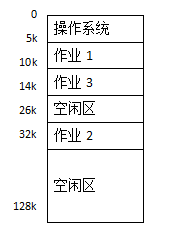为了说明哪些区是空闲的，可以用来装入新作业，必须要有一张空闲区说明表，格式如下：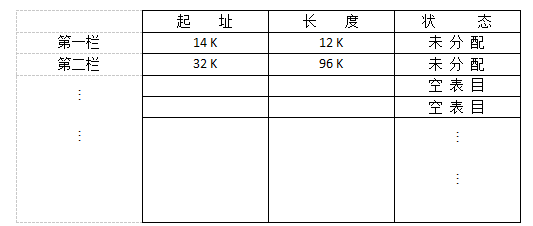起址——指出一个空闲区的主存起始地址。
长度——指出从起始地址开始的一个连续空闲的长度。
状态——有两种状态，一种是“未分配”状态，指出对应的由起址指出的某个长度的区域是空闲区；另一种是“空表目”状态，表示表中对应的登记项目是空白（无效），可用来登记新的空闲区（例如，作业撤离后，它所占的区域就成了空闲区，应找一个“空表目”栏登记归还区的起址和长度且修改状态）。由于分区的个数不定，所以空闲区说明表中应有适量的状态为“空表目”的登记栏目，否则造成表格“溢出”无法登记。
上述的这张说明表的登记情况是按提示：
(1)中的例所装入的三个作业占用的主存区域后填写的。
(2)当有一个新作业要求装入主存时，必须查空闲区说明表，从中找出一个足够大的空闲区。有时找到的空闲区可能大于作业需要量，这时应把原来的空闲区变成两部分：一部分分给作业占用；另一部分又成为一个较小的空闲区。为了尽量减少由于分割造成的空闲区，而尽量保存高地址部分有较大的连续空闲区域，以利于大型作业的装入。为此，在空闲区说明表中，把每个空闲区按其地址顺序登记，即每个后继的空闲区其起始地址总是比前者大。为了方便查找还可使表格“紧缩”，总是让“空表目”栏集中在表格的后部。
(3) 采用最先适应算法（顺序分配算法）分配主存空间。按照作业的需要量，查空闲区说明表，顺序查看登记栏，找到第一个能满足要求的空闲区。当空闲区大于需要量时，一部分用来装入作业，另一部分仍为空闲区登记在空闲区说明表中。由于本实验是模拟主存的分配，所以把主存区分配给作业后并不实际启动装入程序装入作业，而用输出“分配情况”来代替。
(4) 当一个作业执行结束撤离时，作业所占的区域应该归还，归还的区域如果与其它空闲区相邻，则应合成一个较大的空闲区，登记在空闲区说明表中。例如，在提示（1）中列举的情况下，如果作业2撤离，归还所占主存区域时，应与上、下相邻的空闲区一起合成一个大的空闲区登记在空闲区说明表中。归还主存时的回收算法如图4-2。
(5) 请按最先适应算法设计主存分配和回收的程序。然后按（1）中假设主存中已装入三个作业，且形成两个空闲区，确定空闲区说明表的初值。现有一个需要主存量为6K的作业4申请装入主存；然后作业3撤离；再作业2撤离。请你为它们进行主存分配和回收，把空闲区说明表的初值以及每次分配或回收后的变化显示出来或打印出来。

3.（第七章）在分页式管理方式下采用位示图来表示主存分配情况，实现主存空间的分配和回收。
(1)分页式存储器把主存分成大小相等的若干块，作业的信息也按块的大小分页，作业装入主存时可把作业的信息按页分散存放在主存的空闲块中，为了说明主存中哪些块已经被占用，哪些块是尚未分配的空闲块，可用一张位示图来指出。位示图可由若干存储单元来构成，其中每一位与一个物理块对应，用0/1表示对应块为空闲/已占用。
(2)假设某系统的主存被分成大小相等的64块，则位示图可用8个字节来构成，另用一单元记录当前空闲块数。如果已有第0，1，4，5，6，9，11，13，24，31，共10个主存块被占用了，那么位示图情况如下：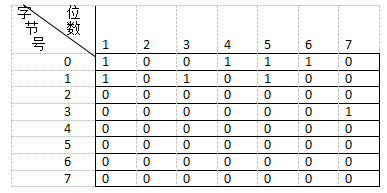(3)当要装入一个作业时，根据作业对主存的需要量，先查当前空闲块数是否能满足作业要求，若不能满足则输出分配不成功。若能满足，则查位示图，找出为“0”的一些位，置上占用标志“1”，从“当前空闲块数”中减去本次占用块数。
按找到的计算出对应的块号，其计算公式为：
块号= j´8+i
其中，j表示找到的是第n个字节，I表示对应的是第n位。
根据分配给作业的块号，为作业建立一张页表，页表格式：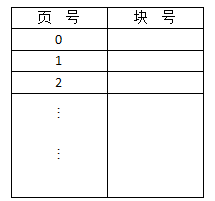(4)当一个作业执行结束，归还主存时，根据该作业的页表可以知道应归还的块号，由块号可计算出在位示图中的对应位置，把对应位的占用标志清成“0”，表示对应的块已成为空闲块。归还的块数加入到当前空闲块数中。由块号计算在位示图中的位置的公式如下：
字节号 j=[块号/8]    （[ ]表示取整）
位数   i={块号/8}     （{ }表示取余）

(5)设计实现主存分配和回收的程序。假定位示图的初始状态如（2）所述，现有一信息量为5页的作业要装入，运行你所设计的分配程序，为作业分配主存且建立页表（格式如（3）所述）。然后假定有另一作业执行结束，它占用的块号为第4，5，6和31块，运行你所设计的回收程序，收回作业归还的主存块。
要求能显示和打印分配或回收前后的位示图和当前空闲块数，对完成一次分配后还要显示或打印为作业建立的页表。

三、方案分析
1.存储管理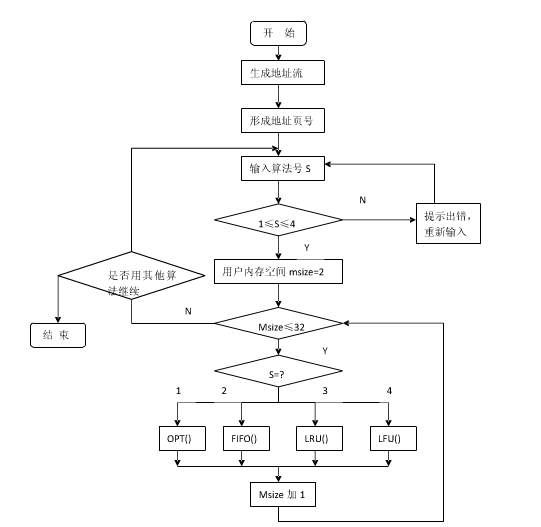2.在可变分区管理方式下采用最先适应算法实现主存分配和实现主存回收。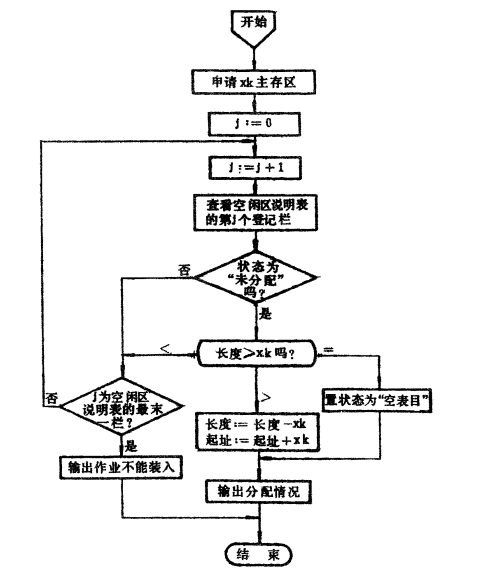3.在分页式管理方式下采用位示图来表示主存分配情况，实现主存空间的分配和回收。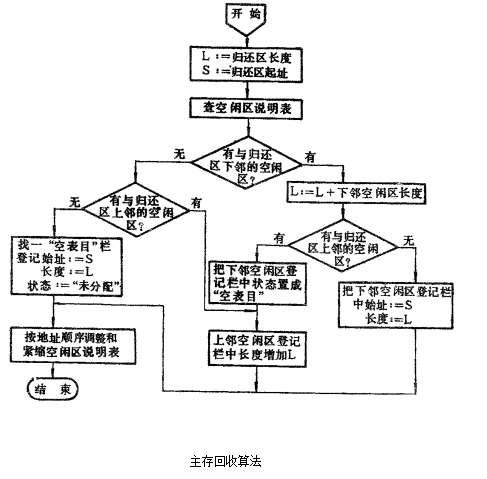四、功能模块实现
1.存储管理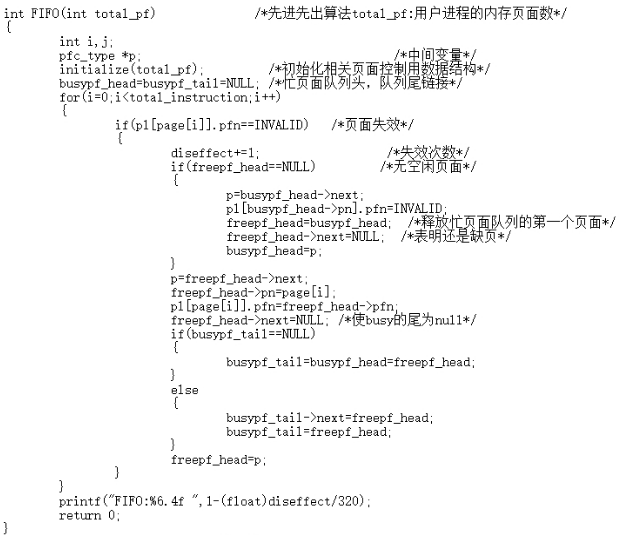2.在可变分区管理方式下采用最先适应算法实现主存分配和实现主存回收。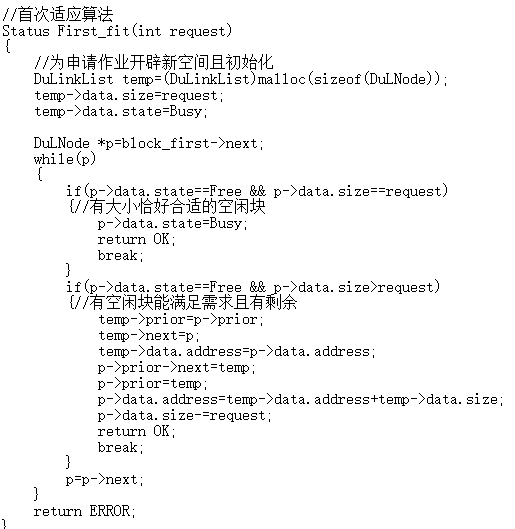3.在分页式管理方式下采用位示图来表示主存分配情况，实现主存空间的分配和回收。
位示图：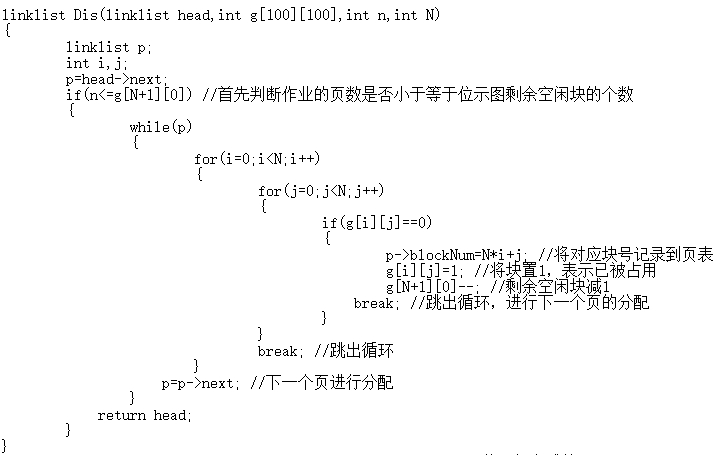链表：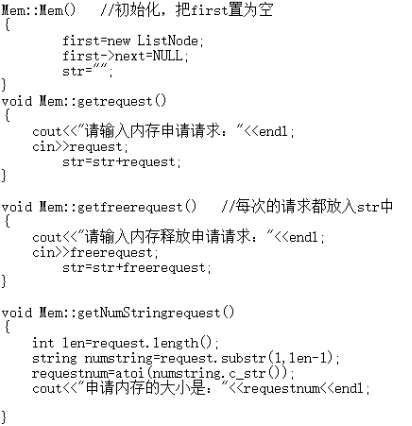五、最终结果分析
1.存储管理：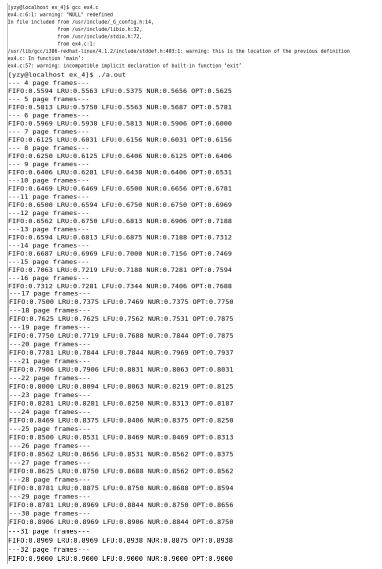2.在可变分区管理方式下采用最先适应算法实现主存分配和实现主存回收。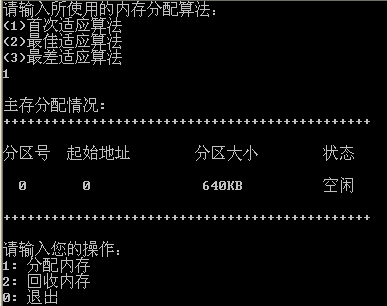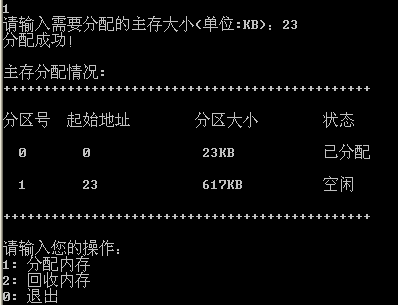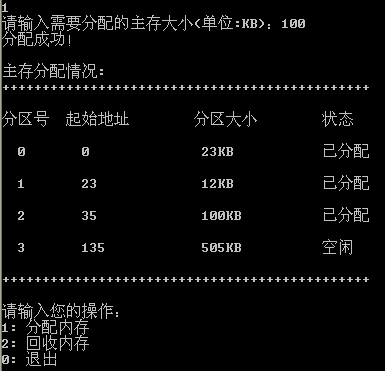3.在分页式管理方式下采用位示图来表示主存分配情况，实现主存空间的分配和回收。
位示图：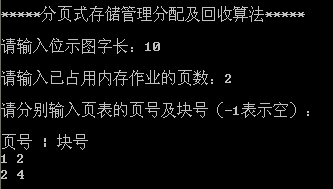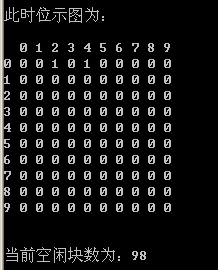链表：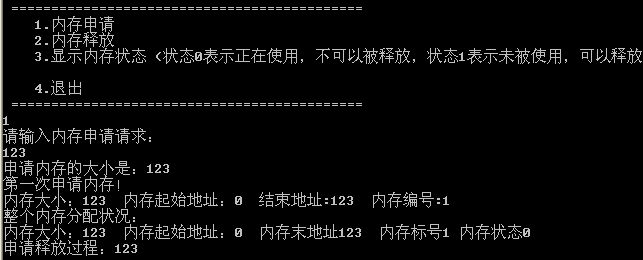六、设计体会
通过切实的实验与分析，我对于存储系统的了解大大的加深了，所有的东西都再是纸上谈兵，从基础的五种算法的实现：先进先出（FIFO）、最近最少使用（LRR）、最佳淘汰（OPT）、最少访问（LFR）、最近最不经常使用（NUR），可以清晰看出操作系统中一些原理性的东西，他的运行，他的工作方式，不只是靠自己对着课本的文字去想想了。
而实验七通过最优、最先、最差三种适应算法来对主存实现分配的过程中，更是深刻的体会到其中的微妙。分页管理中的位示图运行结果出现之后一目了然，链表方式也是方便自如。是让书上不动的文字活了起来。
整个实验下来，无论是翻课本查原理，还是上百度搜代码，都是促进我们学习实践的一大助力，让课堂上一些死板的知识流转在手指之间，跃现在荧屏上面。

七、附录

//1.存储管理。
#define TRUE 1
#define FALSE 0
#define INVALID -1
#define NULL  0
#define  total_instruction 320     /*指令流长*/
#define  total_vp  32               /*虚页长*/
#define  clear_period  50           /*清0周期*/

typedef struct                      /*页面结构*/
{
int pn;      //页号 logic number
int pfn;     //页面框架号 physical frame number
int counter; //计数器
int time;    //时间
}pl_type;

pl_type pl[total_vp];                      /*页面线性结构---指令序列需要使用地址*/

typedef struct pfc_struct                  /*页面控制结构，调度算法的控制结构*/
{
int pn;
int pfn;
struct pfc_struct *next;
}pfc_type;

pfc_type pfc[total_vp], *freepf_head, *busypf_head, *busypf_tail;

int diseffect,  a[total_instruction]; /* a[]为指令序列*/

int page[total_instruction],  offset[total_instruction];/*地址信息*/

int  initialize(int);
int  FIFO(int);
int  LRU(int);
int  LFU(int);
int  NUR(int); //not use recently
int  OPT(int);

int main( )
{
int s,i,j;

srand(10*getpid());                    /*由于每次运行时进程号不同，故可用来作为初始化随机数队列的“种子”*/

s=(float)319*rand( )/32767/32767/2+1;  /*正态分布*/

for(i=0;i<total_instruction;i+=4)        /*产生指令队列*/
{
if(s<0||s>319)
{
printf("When i==%d,Error,s==%d\n",i,s);
exit(0);
}
a[i]=s;                                   /*任选一指令访问点m*/
a[i+1]=a[i]+1;                            /*顺序执行一条指令*/
a[i+2]=(float)a[i]*rand( )/32767/32767/2; /*执行前地址指令m*/
a[i+3]=a[i+2]+1;                          /*顺序执行一条指令*/

s=(float)(318-a[i+2])*rand( )/32767/32767/2+a[i+2]+2;
if((a[i+2]>318)||(s>319))

printf("a[%d+2],a number which is :%d and s==%d\n",i,a[i+2],s);

}
for (i=0;i<total_instruction;i++) /*将指令序列变换成页地址流*/
{
page[i]=a[i]/10;
offset[i]=a[i]%10;
}
for(i=4;i<=32;i++)   /*用户内存工作区从4个页面到32个页面*/
{
printf("---%2d page frames---\n",i);
FIFO(i);
LRU(i);
LFU(i);
NUR(i);
OPT(i);

}
return 0;
}

/*初始化相关数据结构 total_pf表示内存的块数 */

int initialize(int total_pf)
{
int i;
diseffect=0;
for(i=0;i<total_vp;i++)
{

pl[i].pfn=INVALID;       /*置页面控制结构中的页号，页面为空*/
pl[i].counter=0;         /*页面控制结构中的访问次数为0*/
pl[i].time=-1;           /*访问的时间*/
}

for(i=0;i<total_pf-1;i++)	/*建立pfc[i-1]和pfc[i]之间的链接*/
{
pfc[i].next=&pfc[i+1];
pfc[i].pfn=i;
}

pfc[total_pf-1].next=NULL;
pfc[total_pf-1].pfn=total_pf-1;
freepf_head=&pfc;         /*空页面队列的头指针为pfc*/
return 0;
}

int FIFO(int total_pf)              /*先进先出算法total_pf:用户进程的内存页面数*/
{
int i,j;
pfc_type *p;					/*中间变量*/
initialize(total_pf);         /*初始化相关页面控制用数据结构*/
busypf_head=busypf_tail=NULL; /*忙页面队列头，队列尾链接*/
for(i=0;i<total_instruction;i++)
{
if(pl[page[i]].pfn==INVALID)   /*页面失效*/
{
diseffect+=1;                  /*失效次数*/
if(freepf_head==NULL)         /*无空闲页面*/
{
p=busypf_head->next;
pl[busypf_head->pn].pfn=INVALID;
freepf_head=busypf_head;  /*释放忙页面队列的第一个页面*/
freepf_head->next=NULL;  /*表明还是缺页*/
busypf_head=p;
}
p=freepf_head->next;
freepf_head->pn=page[i];
pl[page[i]].pfn=freepf_head->pfn;
freepf_head->next=NULL; /*使busy的尾为null*/
if(busypf_tail==NULL)
{
busypf_tail=busypf_head=freepf_head;
}
else
{
busypf_tail->next=freepf_head;
busypf_tail=freepf_head;
}
freepf_head=p;
}
}
printf("FIFO:%6.4f ",1-(float)diseffect/320);
return 0;
}
int LRU (int total_pf)       /*最近最久未使用算法least recently used*/
{
int min,minj,i,j,present_time; /*minj为最小值下标*/
initialize(total_pf);
present_time=0;
for(i=0;i<total_instruction;i++)
{
if(pl[page[i]].pfn==INVALID)             /*页面失效*/
{
diseffect++;
if(freepf_head==NULL)              /*无空闲页面*/
{
min=32767;						/*设置最大值*/
for(j=0;j<total_vp;j++)            /*找出time的最小值*/
{
if(min>pl[j].time&&pl[j].pfn!=INVALID)
{
min=pl[j].time;
minj=j;
}
}
freepf_head=&pfc[pl[minj].pfn];   //腾出一个单元
pl[minj].pfn=INVALID;
pl[minj].time=0;
freepf_head->next=NULL;
}
pl[page[i]].pfn=freepf_head->pfn;   //有空闲页面,改为有效
pl[page[i]].time=present_time;
freepf_head=freepf_head->next;      //减少一个free 页面
}
else
{
pl[page[i]].time=present_time;        //命中则增加该单元的访问次数
present_time++;
}
}
printf("LRU:%6.4f ",1-(float)diseffect/320);
return 0;
}

int NUR(int  total_pf )                  /*最近未使用算法Not Used recently count表示*/
{
int i,j,dp,cont_flag,old_dp;
pfc_type *t;
initialize(total_pf);
dp=0;

for(i=0;i<total_instruction;i++)
{
if (pl[page[i]].pfn==INVALID)         /*页面失效*/
{
diseffect++;
if(freepf_head==NULL)               /*无空闲页面*/
{
cont_flag=TRUE;
old_dp=dp;

while(cont_flag)
{

if(pl[dp].counter==0&&pl[dp].pfn!=INVALID)
cont_flag=FALSE;
else
{
dp++;
if(dp==total_vp)
dp=0;
if(dp==old_dp)
for(j=0;j<total_vp;j++)
pl[j].counter=0;
}
}
freepf_head=&pfc[pl[dp].pfn];
pl[dp].pfn=INVALID;
freepf_head->next=NULL;
}

pl[page[i]].pfn=freepf_head->pfn;

freepf_head->pn=page[i];

freepf_head=freepf_head->next;
}
else
pl[page[i]].counter=1;
if(i%clear_period==0)
for(j=0;j<total_vp;j++)
pl[j].counter=0;
}
printf("NUR:%6.4f ",1-(float)diseffect/320);
return 0;
}

int OPT(int total_pf)       /*最佳置换算法*/
{
int i,j, max,maxpage,d,dist[total_vp];
pfc_type *t;
initialize(total_pf);
for(i=0;i<total_instruction;i++)
{
if(pl[page[i]].pfn==INVALID)      /*页面失效*/
{
diseffect++;
if(freepf_head==NULL)         /*无空闲页面*/
{
for(j=0;j<total_vp;j++)
{
if(pl[j].pfn!=INVALID)
dist[j]=32767;
else
dist[j]=0;
}
for(j=0;j<total_vp;j++)
{
if((pl[j].pfn!=INVALID)&&(dist[j]==32767))
{
dist[j]=j;
}
}
max=0;
for(j=0;j<total_vp;j++)
if(max<dist[j])
{
max=dist[j];
maxpage=j;
}
freepf_head=&pfc[pl[maxpage].pfn];
freepf_head->next=NULL;
pl[maxpage].pfn=INVALID;
}
pl[page[i]].pfn=freepf_head->pfn;
freepf_head=freepf_head->next;
}
}
printf("OPT:%6.4f\n",1-(float)diseffect/320);
return 0;
}
/*该算法时根据已知的预测未知的，least frequency  Used是最不经常使用置换法*/
int  LFU(int total_pf)
{
int i,j,min,minpage;
pfc_type *t;
initialize(total_pf);
for(i=0;i<total_instruction;i++)
{
if(pl[page[i]].pfn==INVALID)      /*页面失效*/
{
diseffect++;
if(freepf_head==NULL)          /*无空闲页面*/
{
min=32767;
/*获取counter的使用用频率最小的内存*/
for(j=0;j<total_vp;j++)
{
if(min>pl[j].counter&&pl[j].pfn!=INVALID)
{
min=pl[j].counter;
minpage=j;
}
}
freepf_head=&pfc[pl[minpage].pfn];
pl[minpage].pfn=INVALID;
pl[minpage].counter=0;
freepf_head->next=NULL;
}
pl[page[i]].pfn=freepf_head->pfn;   //有空闲页面,改为有效
pl[page[i]].counter++;
freepf_head=freepf_head->next;      //减少一个free 页面
}
else
{
pl[page[i]].counter;
pl[page[i]].counter=pl[page[i]].counter+1;
}
}
printf("LFU:%6.4f ",1-(float)diseffect/320);
return 0;
}

//2.在可变分区管理方式下采用最先适应算法实现主存分配和实现主存回收。
#include<iostream>
#include<stdlib.h>
using namespace std;

#define Free 0 //空闲状态
#define Busy 1 //已用状态
#define OK 1    //完成
#define ERROR 0 //出错
#define MAX_length 640 //最大内存空间为640KB
typedef int Status;
int flag;

typedef struct freearea//定义一个空闲区说明表结构
{
long size;   //分区大小
long address; //分区地址
int state;   //状态
}ElemType;

// 线性表的双向链表存储结构
typedef struct DuLNode
{
ElemType data;
struct DuLNode *prior; //前趋指针
struct DuLNode *next;  //后继指针
}

DuLNode,*DuLinkList;
DuLinkList block_first; //头结点
DuLinkList block_last;  //尾结点
Status alloc(int);//内存分配
Status free(int); //内存回收
Status First_fit(int);//首次适应算法
Status Best_fit(int); //最佳适应算法
Status Worst_fit(int); //最差适应算法
void show();//查看分配
Status Initblock();//开创空间表

Status Initblock()//开创带头结点的内存空间链表
{
block_first=(DuLinkList)malloc(sizeof(DuLNode));
block_last=(DuLinkList)malloc(sizeof(DuLNode));
block_first->prior=NULL;
block_first->next=block_last;
block_last->prior=block_first;
block_last->next=NULL;
block_last->data.address=0;
block_last->data.size=MAX_length;
block_last->data.state=Free;
return OK;
}

//分配主存
Status alloc(int ch)
{
int request = 0;
cout<<"请输入需要分配的主存大小(单位:KB)：";
cin>>request;
if(request<0 ||request==0)
{
cout<<"分配大小不合适，请重试！"<<endl;
return ERROR;
}

if(ch==2) //选择最佳适应算法
{
if(Best_fit(request)==OK) cout<<"分配成功！"<<endl;
else cout<<"内存不足，分配失败！"<<endl;
return OK;
}
if(ch==3) //选择最差适应算法
{
if(Worst_fit(request)==OK) cout<<"分配成功！"<<endl;
else cout<<"内存不足，分配失败！"<<endl;
return OK;
}
else //默认首次适应算法
{
if(First_fit(request)==OK) cout<<"分配成功！"<<endl;
else cout<<"内存不足，分配失败！"<<endl;
return OK;
}
}

//首次适应算法
Status First_fit(int request)
{
//为申请作业开辟新空间且初始化
DuLinkList temp=(DuLinkList)malloc(sizeof(DuLNode));
temp->data.size=request;
temp->data.state=Busy;

DuLNode *p=block_first->next;
while(p)
{
if(p->data.state==Free && p->data.size==request)
{//有大小恰好合适的空闲块
p->data.state=Busy;
return OK;
break;
}
if(p->data.state==Free && p->data.size>request)
{//有空闲块能满足需求且有剩余
temp->prior=p->prior;
temp->next=p;
temp->data.address=p->data.address;
p->prior->next=temp;
p->prior=temp;
p->data.address=temp->data.address+temp->data.size;
p->data.size-=request;
return OK;
break;
}
p=p->next;
}
return ERROR;
}

//最佳适应算法
Status Best_fit(int request)
{
int ch; //记录最小剩余空间
DuLinkList temp=(DuLinkList)malloc(sizeof(DuLNode));
temp->data.size=request;
temp->data.state=Busy;
DuLNode *p=block_first->next;
DuLNode *q=NULL; //记录最佳插入位置

while(p) //初始化最小空间和最佳位置
{
if(p->data.state==Free && (p->data.size>=request) )
{
if(q==NULL)
{
q=p;
ch=p->data.size-request;
}
else if(q->data.size > p->data.size)
{
q=p;
ch=p->data.size-request;
}
}
p=p->next;
}

if(q==NULL) return ERROR;//没有找到空闲块
else if(q->data.size==request)
{
q->data.state=Busy;
return OK;
}
else
{
temp->prior=q->prior;
temp->next=q;
temp->data.address=q->data.address;
q->prior->next=temp;
q->prior=temp;
q->data.address+=request;
q->data.size=ch;
return OK;
}
return OK;
}

//最差适应算法
Status Worst_fit(int request)
{
int ch; //记录最大剩余空间
DuLinkList temp=(DuLinkList)malloc(sizeof(DuLNode));
temp->data.size=request;
temp->data.state=Busy;
DuLNode *p=block_first->next;
DuLNode *q=NULL; //记录最佳插入位置

while(p) //初始化最大空间和最佳位置
{
if(p->data.state==Free && (p->data.size>=request) )
{
if(q==NULL)
{
q=p;
ch=p->data.size-request;
}
else if(q->data.size < p->data.size)
{
q=p;
ch=p->data.size-request;
}
}
p=p->next;
}

if(q==NULL) return ERROR;//没有找到空闲块
else if(q->data.size==request)
{
q->data.state=Busy;
return OK;
}
else
{
temp->prior=q->prior;
temp->next=q;
temp->data.address=q->data.address;
q->prior->next=temp;
q->prior=temp;
q->data.address+=request;
q->data.size=ch;
return OK;
}
return OK;
}

//主存回收
Status free(int flag)
{
DuLNode *p=block_first;
for(int i= 0; i <= flag; i++)
if(p!=NULL)
p=p->next;
else
return ERROR;

p->data.state=Free;
if(p->prior!=block_first && p->prior->data.state==Free)//与前面的空闲块相连
{
p->prior->data.size+=p->data.size;
p->prior->next=p->next;
p->next->prior=p->prior;
p=p->prior;
}
if(p->next!=block_last && p->next->data.state==Free)//与后面的空闲块相连
{
p->data.size+=p->next->data.size;
p->next->next->prior=p;
p->next=p->next->next;
}
if(p->next==block_last && p->next->data.state==Free)//与最后的空闲块相连
{
p->data.size+=p->next->data.size;
p->next=NULL;
}

return OK;
}

//显示主存分配情况
void show()
{
int flag = 0;
cout<<"\n主存分配情况:\n";
cout<<"++++++++++++++++++++++++++++++++++++++++++++++\n\n";
DuLNode *p=block_first->next;
cout<<"分区号\t起始地址\t分区大小\t状态\n\n";
while(p)
{
cout<<"  "<<flag++<<"\t";
cout<<"  "<<p->data.address<<"\t\t";
cout<<" "<<p->data.size<<"KB\t\t";
if(p->data.state==Free) cout<<"空闲\n\n";
else cout<<"已分配\n\n";
p=p->next;
}
cout<<"++++++++++++++++++++++++++++++++++++++++++++++\n\n";
}

//主函数
void main()
{
int ch;//算法选择标记
cout<<"请输入所使用的内存分配算法：\n";
cout<<"(1)首次适应算法\n(2)最佳适应算法\n(3)最差适应算法\n";

cin>>ch;
while(ch<1||ch>3)
{
cout<<"输入错误，请重新输入所使用的内存分配算法：\n";
cin>>ch;
}
Initblock(); //开创空间表
int choice;  //操作选择标记
while(1)
{
show();
cout<<"请输入您的操作：";
cout<<"\n1: 分配内存\n2: 回收内存\n0: 退出\n";

cin>>choice;
if(choice==1) alloc(ch); // 分配内存
else if(choice==2)  // 内存回收
{
int flag;
cout<<"请输入您要释放的分区号：";
cin>>flag;
free(flag);
}
else if(choice==0) break; //退出
else //输入操作有误
{
cout<<"输入有误，请重试！"<<endl;
continue;
}
}
}

//3.在分页式管理方式下采用位示图来表示主存分配情况，实现主存空间的分配和回收。
//（1）链表方式：
#include <iostream>
#include <string>
using namespace std;

struct ListNode  //链表的节点
{
int begin;
int end;
int size;
int num;
bool freeable;
ListNode *next;
};

typedef ListNode *  ListPtr;

class Mem
{
public:
Mem();
void getNumStringrequest();
void getNumStringfree();
void getrequest();
void getfreerequest();
// int getNum();
//void Insert();
//void Delete();
void Print();
void freemem();
void getmem();
private:
ListPtr first;
string request;
int requestnum;
int freenum;
string freerequest;
string str;
};

Mem::Mem()   //初始化，把first置为空
{
first=new ListNode;
first->next=NULL;
str="";
}
void Mem::getrequest()
{
cout<<"请输入内存申请请求："<<endl;
cin>>request;
str=str+request;
}

void Mem::getfreerequest()   //每次的请求都放入str中
{
cout<<"请输入内存释放申请请求："<<endl;
cin>>freerequest;
str=str+freerequest;
}

void Mem::getNumStringrequest()
{
int len=request.length();
string numstring=request.substr(1,len-1);
requestnum=atoi(numstring.c_str());
cout<<"申请内存的大小是："<<requestnum<<endl;

}
void Mem::getNumStringfree()   //使用atoi函数将char *（string通过strin.c_str())可以得到char *类型
{
int len=freerequest.length();
string freestring=freerequest.substr(5,len-2);
freenum=atoi(freestring.c_str());
cout<<"释放内存的起始地址是："<<freenum<<endl;
}

void Mem::freemem()
{
ListNode *p=first;
while(p->next!=NULL)
{
if(p->next->begin==freenum&&p->next->freeable==false)
{
cout<<"释放内存！"<<p->next->num<<endl;
p->next->freeable=true;
}
ListNode *q=p->next;
if(q->freeable==true)   //采用向前合并的原则
{

if(q->next!=NULL&&q->next->freeable==true&&p->freeable==true)  //前后均为空
{
cout<<"释放内存的前后都为空，因此将三块内存合并！"<<endl;  //3块内存合并到最前面一块
p->size=p->size+q->size+q->next->size;
p->freeable=true;
p->end=p->begin+p->size;
ListNode *k=q->next;
p->next=k->next;
delete q;
delete k;
}
else if(p->freeable==true&&q->next!=NULL&&q->next->freeable==false)  //前为空，后面不空
{
cout<<"释放内存的前一块是空的，合并"<<endl;
p->size=p->size+q->size;
p->freeable=true;
p->end=p->begin+p->size;
p->next=q->next;
delete q;
}

else if(p->freeable==false&&q->freeable==true&&q->next->freeable==true)  //前面空后面不空
{
cout<<"释放内存的后一块是空的，合并！"<<endl;
ListNode * k=q->next;
q->size=q->size+k->size;
q->freeable=true;
q->next=k->next;
q->end=q->begin+q->size;
delete k;
}

}

p=p->next;
}

}

void Mem::getmem()
{
ListNode * p=first->next;
ListNode *q;
if(p==NULL)  //第一次申请内存
{
cout<<"第一次申请内存！"<<endl;
q=new ListNode;
q->begin=0;
q->freeable=false;
q->size=requestnum;
q->end=q->begin+q->size;
q->num=1;
first->next=q;
cout<<"内存大小："<<q->size<<"  内存起始地址："<<q->begin<<"  结束地址:"<<q->end<<"  内存编号:"<<q->num<<endl;
q->next=NULL;
}
else  //前面有释放的内存。不向后面才查找
{
bool find=false;
p=first;
while(p->next!=NULL&&find!=true)
{
if(p->next!=NULL&&p->next->size>requestnum&&p->next->freeable==true)
{
cout<<"找到空的内存："<<endl;
cout<<"内存大小："<<p->next->size<<"  内存起始地址："<<p->next->begin<<"  结束地址:"<<p->next->end<<"  内存编号:"<<p->next->num<<"  内存状态："<<p->freeable<<endl;
cout<<"将大内存分割："<<endl;
ListNode *temp=p->next;
ListNode *k=new ListNode;
// k=p->next;
k->begin=p->next->begin;
k->freeable=false;
k->size=requestnum;
k->end=p->next->begin+k->size;
k->num=temp->num;
p->next=k;

ListNode *l=new ListNode;

l->begin=k->end+1;
l->size=temp->size-k->size;
l->end=l->begin+l->size;
l->freeable=true;
l->num=k->num;
l->next=temp->next;

k->next=l;
find=true;

delete temp;
}
p=p->next;
}

if(false==find)  //前面没找到合适的内存，因此开辟一块新的内存
{
p=first;
cout<<"开辟一块新的内存！"<<endl;
ListNode *q=new ListNode;
while(p->next!=NULL)
p=p->next;
q->begin=p->end+1;
q->end=q->begin+requestnum;
q->freeable=false;
q->num=p->num+1;
q->size=requestnum;
p->next=q;
q->next=NULL;

}
}
}

void Mem::Print()
{
cout<<"整个内存分配状况："<<endl;
ListNode *p=first->next;
while(p!=NULL)
{

cout<<"内存大小："<<p->size<<"  内存起始地址："<<p->begin<<"  内存末地址"<<p->end<<"  内存标号"<<p->num<<" 内存状态"<<p->freeable<<endl;
p=p->next;
}
cout<<"申请释放过程："<<str<<endl;
}
int main()
{
Mem mem;
string str="";
char quit='n';
while(quit!='Y'&&quit!='y')  //采用while一直循环实行模拟内存的申请与释放
{
int chose;
cout<<" ============================================"<<endl;
cout<<"    1.内存申请     "<<endl;
cout<<"    2.内存释放     "<<endl;
cout<<"    3.显示内存状态 (状态0表示正在使用，不可以被释放，状态1表示未被使用，可以释放"<<endl;
cout<<"    4.退出          "<<endl;
cout<<" ============================================"<<endl;
cin>>chose;
switch(chose)
{
case 1:
mem.getrequest();
mem.getNumStringrequest();
mem.getmem();
mem.Print();
break;
case 2:
mem.getfreerequest();
mem.getNumStringfree();
mem.freemem();
mem.Print();
break;
case 3:
mem.Print();
case 4:   //一旦用户选择退出，那么置quit为YES退出程序
quit='y';
default:
break;
}
}
/*mem.getrequest();
mem.getNumStringrequest();
mem.getmem();
mem.Print();
mem.freemem();
mem.Print();
mem.getfreerequest();
mem.getNumStringfree();
*/
return 0;

}

//（2）位示图：
#include <stdlib.h>
#include <stdio.h>
typedef int datatype;

typedef struct node
{
datatype pageNum,blockNum;
struct node *next;
}linknode;

typedef linknode *linklist;

linklist creatlinklist(int n)/*尾插法创建带头结点的单链表*/
{
linklist head,r,s;
int x,y,i=0;
head=r=(linklist)malloc(sizeof(linknode));
printf("\n请分别输入页表的页号及块号（-1表示空）：\n");
printf("\n页号 | 块号\n");
while (i<n)
{
scanf("%d %d",&x,&y);
s=(linklist)malloc(sizeof(linknode));
s->pageNum=x;
s->blockNum=y;
r->next=s;
r=s;
i++;
}
r->next=NULL;
return head;
}

void init(int g,int N)/*初始化位示图，将值全置为零，0表示空闲状态*/
{
int i,j;
for(i=0;i<100;i++)
{
for(j=0;j<100;j++)
{
g[i][j]=0; //全置为零
}
}
g[N+1]=N*N; //在数组最后一个数的后面设置一个空间用来存放剩余空闲块数
}

linklist Init(linklist head,int g,int n,int N)
{
linklist p;
int i,j;
p=head->next;
if(n<=g[N+1]) //首先判断作业的页数是否小于等于位示图剩余空闲块的个数
{
while(p)
{
i=p->blockNum/N;
j=p->blockNum%N;
g[i][j]=1;
g[N+1]--;
p=p->next;
}
}
return head;

}
printStr(int g,int N)/*打印位示图*/
{
int i,j;
printf("\n此时位示图为：\n");
printf("\n ");
for(i=0;i<N;i++)
{
printf(" ");
printf("%d",i);
}
printf("\n");
for(i=0;i<N;i++)
{
printf("%d",i);
for(j=0;j<N;j++)
{
printf(" ");
printf("%d",g[i][j]);
}
printf("\n");
}
printf("\n");
}
void print(linklist head)/*输出带头结点的单链表*/
{
linklist p;
p=head->next;
printf("\n该页表为:\n");
printf("\n");
printf("\n         页号 | 块号\n");
while(p)
{
printf("%11d%7d\n",p->pageNum,p->blockNum);
p=p->next;
}

linklist Dis(linklist head,int g,int n,int N)
{
linklist p;
int i,j;
p=head->next;
if(n<=g[N+1]) //首先判断作业的页数是否小于等于位示图剩余空闲块的个数
{
while(p)
{
for(i=0;i<N;i++)
{
for(j=0;j<N;j++)
{
if(g[i][j]==0)
{
p->blockNum=N*i+j; //将对应块号记录到页表
g[i][j]=1; //将块置1，表示已被占用
g[N+1]--; //剩余空闲块减1
break; //跳出循环，进行下一个页的分配
}
}
break; //跳出循环
}
p=p->next; //下一个页进行分配
}
return head;
}
}
linklist Recy(linklist head,int g,int n,int N)/*回收已经完成的页*/
{
int i,j;
linklist p;
p=head->next;
while(p&&p->pageNum!=n) //找出要回收的页号
{
p=p->next;
}

if(p) //找到
{
i=p->blockNum/N;
j=p->blockNum%N;
g[i][j]=0; //将该块置0，表空闲状态
g[N+1]++;
p->blockNum=-1; //页表中对应的块号为空，置成-1
}
return head;
}
void main()
{
int m,n,N;
int x,y,a,b,t;
int graph;
linklist head,Head;
printf("\n*****分页式存储管理分配及回收算法*****\n");
printf("\n请输入位示图字长：");
scanf("%d",&N);
printf("\n请输入已占用内存作业的页数：");
scanf("%d",&m);
head=creatlinklist(m);
init(graph,N);
head=Init(head,graph,m,N);
printStr(graph,N);
printf("\n当前空闲块数为：%d",graph[N+1]);
printf("\n\n现在进行作业分配：\n");
printf("\n请输入需要分配的作业的页数：");
scanf("%d",&n);
Head=creatlinklist(n);
Head=Dis(Head,graph,n,N);
print(Head);
printStr(graph,N);
printf("\n当前空闲块数为：%d",graph[N+1]);
printf("\n\n您是否想回收已完成的页，“是”请按1，“否”请按0：");
scanf("%d",&x);
if(x) //判断是否要回收
{
printf("\n请输入您要回收的页号：");
scanf("%d %d %d %d",&y,&a,&b,&t);
head=Recy(head,graph,y,N);
head=Recy(head,graph,a,N);
head=Recy(head,graph,b,N);
head=Recy(head,graph,t,N);
printStr(graph,N);
}
printf("\n当前空闲块数为：%d",graph[N+1]);
printf("\n");
}


展开全文设计
• 设计一个虚拟存储区和内存工作区，并使用下述算法计算访问命中率。 1、最佳淘汰算法（OPT） 2、先进先出的算法（FIFO） 3、最近最久未使用算法（LRU） 4、最不经常使用算法（LFU） 5、最近未使用算法（NUR） 命中率...
• #include <iostream> using namespace std; int main() { int num; //物理块 int count; //要输入的页面数量 int Page_Num; //页面号 int empt=0; // 标记空框 int tianman = 1; //第一次填满标记 ... /
#include <iostream>
using namespace std;

int main()
{
int num;  //物理块
int count; //要输入的页面数量
int Page_Num;  //页面号
int empt=0; // 标记空框
int tianman = 1; //第一次填满标记
int Page_fault_num = 0; //缺页数量
float Page_fault_rate = 0; //缺页率
int state = 0;  //标记有没有相同的页
int biaoji = 0; //标记替换第几个数
int M = { 0 };
cout << "请输入信息块数目：";
cin >> num;
cout << "输入总共的页面数：";
cin >> count;
cout << "请输入页面号：";

for (int i = 0; i < count; i++)
{
state = 0;
cin >> Page_Num;
cout << Page_Num;

if (i == 0) {   //第一个可以直接放入
M[i] = Page_Num;
cout << "*";
Page_fault_num++;
}
else {

for (int j = tianman; j < num; j++)  //判断有几个块从未被填充过
{
if (M[j] == 0) {   //标记第一个空框
empt = j;
break;
}
}

if (tianman > num)
{
for (int j = 0; j < num; j++)  //判断有没有一样的
{
if (M[j] == Page_Num)    //有一样的，state加标记
{
state++;
}
}
}

else
{
for (int j = 0; j < tianman; j++)  //判断有没有一样的
{
if (M[j] == Page_Num)    //有一样的，state加标记
{
state++;
}
}
}

if (state == 0)  //没有一样的，换掉某位置数组
{
if (tianman < num)  //没被填满的时候填充
{
M[empt] = Page_Num;
cout << "*";
tianman++;
Page_fault_num++;
}
else {      //曾经被填满过
M[biaoji] = Page_Num;
biaoji++;
cout << "*";
Page_fault_num++;
}

}
else {   //有相同的输出“-”
cout << "-";
}
}
if (biaoji == num)  //置换为第num块后回到第一块
{
biaoji = 0;
}
for (int q = 0; q < num; q++)
{
cout << M[q];
}
cout << endl;
}
Page_fault_rate = Page_fault_num * 1.0 / count;
cout << "缺页率为：" << Page_fault_rate;
}



展开全文• 1.通过编写和调试存储管理的模拟程序以加深对存储管理方案的理解。熟悉虚存管理的各种页面淘汰算法 2.通过编写和调试地址转换过程的模拟程序以加强对地址转换过程的了解。 二．实验要求 实验程序由以下三大部分...
• 1. 设计思路 使用c++栈函数 最近使用的放在栈顶，最久未使用的处于栈底。 判断栈内是否为空（判断当前页框中有没有页面） 为空->直接入栈 不为空->通过辅助栈对主栈内容进行遍历 有相同的，将原栈中的页面...
1. 设计思路
使用c++栈函数
最近使用的放在栈顶，最久未使用的处于栈底。

判断栈内是否为空（判断当前页框中有没有页面）
为空->直接入栈
不为空->通过辅助栈对主栈内容进行遍历
有相同的，将原栈中的页面删除，将新进的页面放在栈顶，从而确保最近使用过的页面处于栈顶
没有相同的，将栈底元素删除，将新进页面放在栈顶，从而将最久未使用的删除并确保最近使用过的页面处于栈顶

2. 代码
/*最近最少使用LRU
* 将最近使用的放在栈顶
*/
#include <iostream>
#include<stack>
#include<vector>
using namespace std;

int main()
{
int physical_block_num; // 物理块数量
int page_num; //页面数
int page_no;  //页面号
stack<int, vector<int>>s1;  //主栈
stack<int, vector<int>>s2;  //辅助栈
cout << "请输入物理块数量：";
cin >> physical_block_num;
cout << "请输入页面数量：";
cin >> page_num;
for (int i = 0; i < page_num; i++)
{
int state = 0;  //标记栈中有没有相同的
cin >> page_no;
if (s1.empty())  //为空直接入栈
{
s1.push(page_no);
cout << "*";
}
else {
while (!s1.empty())
{
if (page_no == s1.top()) {   //有相同的
cout << "-";
s1.pop();  //把相同的丢掉
while (!s2.empty())
{
s1.push(s2.top()); //把s2中的放回来
s2.pop();
}
s1.push(page_no); //把最新加入的放到栈顶，保证最新使用过的在上面
state = 1;
break;
}
else {
s2.push(s1.top());   //把s1中的元素放入s2中
s1.pop();  //把换到s2中的，删除
}
}
if (state == 0) {  //如果没有相同的，s1中的元素会被全部换到s2中
if (s2.size() == physical_block_num)  //判断需不需要把原来栈中的内容删除
{
s2.pop();  //先把s2中的第一个删除，第一个是最久未使用的
while (!s2.empty()) {
s1.push(s2.top());  //把s2中的其他元素放入s1中
s2.pop();           //把s2中放入s1中的元素删除
}
s1.push(page_no);  //把最新添加进来的放在最上面
cout << "*";
}
else {
while (!s2.empty()) {
s1.push(s2.top());  //把s2中的其他元素放入s1中
s2.pop();  //把s2中放入s1中的元素删除
}
s1.push(page_no);  //把最新添加进来的放在最上面
cout << "*";
}
}

}
while (!s1.empty())
{
cout << s1.top();
s2.push(s1.top());
s1.pop();
}
while (!s2.empty())
{
s1.push(s2.top());
s2.pop();
}
cout << endl;
}
}
/*test data
3
20
9 2 3 4 2 5 2 6 4 5 2 5 4 3 4 2 3 9 2 3
*/


3. 测试数据
3
20
9 2 3 4 2 5 2 6 4 5 2 5 4 3 4 2 3 9 2 3展开全文• 操作系统虚拟存储管理实验 开辟一块内存空间，作为模拟内存(malloc) 空间大小为2^14字节 假设系统的页面大小为256字节，每个页表项占4个字节(系统的物理页面数为2^6,每个页表正好占一个页面) 用位图刻画内存页面的...
• 输入进程数，创建随机进程，按照从0开始的时间量，根据到达时刻和所需空间，计算工作分区表和空闲分区表，直到所有进程完成为止。
• 存储管理的主要功能之一是合理地分配空间。请求页式管理是一种常用的虚拟存储管理技术。...本实验的目的是通过请求页式存储管理中页面置换算法模拟设计，了解虚拟存储技术的特点，掌握请求页式存储管理的页面置换算法。
• 计算机操作系统内存管理功能实现存储空间的分配与回收。当用户提出申请存储器空间时，存储管理必须根据申请者的要求，按一定的策略分析主存空间的使用情况，找出足够的空闲区域分配给申请者。当作业撤离或主动归还...
• 操作系统实验 存储管理 呵呵 我们地实验 交上去地 是doc格式地
• 三、实验内容 （1） 通过随机数产生一个指令序列，共320条指令。指令的地址按下述原则生成： 1. 50%的指令是顺序执行的； 2. 25%的指令是均匀分布在前地址部分； 3. 25%的指令是均匀分布在后地址部分； 具体的实施...
• 1、最近最久未使用置换算法LRU是根据页面调入内存后的使用情况进行决策的，它是利用“最近的过去”作为“最近的将来”的近似，选择最近最久未使用的页面予以淘汰。2、最佳淘汰算法OPT：也称最佳置换算法。...
• 理解操作系统存储管理原理 操作系统的发展使得系统完成了大部分的内存管理工作。对于程序员而言，这些内存管理的过程完全透明不可见。因此，程序员开发时从不关心系统如何为自己分配内存，而且永远认为系统可以分配...
• 想知道操作系统是怎么管理内存的吗？ malloc是怎么实现的呢？ 通过自己的动手实践，这里为大家提供了一个内存管理和分配的模型——存储桶模型！ 压缩包内包含源代码（有注释）和说明文档。 在读者认真阅读和理解之后...
• 教育资料 教育资料 操作系统实验报告 实验序号 7 实验项目名称 Linux 存储管理操作实践 学号 姓名 专业班 实验地点 指导教师 实验时间 一实验目的及要求 通过本实验的学习使学生掌握 Linux 存储管理相关操作的基本......